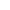Top | Introduction | Members | Activities | Call for Paper | Link |Japanese## "A study on the security of symmetric key cipher"

 ```The value of the information came to be reviewed by an explosive spread of the Internet in recent years. Therefore, the problem concerning information security like tapping, the falsification, and the unlawful computer access etc. of information has become important in the network society of today. The cipher is known as a technology that does the basis in information security. The cipher is a technology that makes information to a scramble, and can prevent the leak to others. There is a symmetric key block cipher in one of such the encryption technologies. The symmetric key block cipher is a cipher to which it is composed of simple operations of exclusive-OR, the additive, the subtraction, multiplication, and the cyclic shift, etc , and data is stirred with the common key by repeating a simple encryption function. The symmetric key block cipher computes at very compact and high speed compared with the public key cryptosystem based on the number theory . Therefore, the symmetric key block cipher is used with the equipment with low-speed CPU such as IC cards and mobile phones and to encrypt a large amount of data. The security of the symmetric key block cipher is decided by the number of rounds that can be attacked more efficiently than the exhaustive search. ``Attack to n rounds possible'' means that it is possible to cracked up to n rounds earlier than the exhaustive search. In this paper, we evaluate the security of the algorithm that is called RC6 in the symmetric key block cipher from which various algorithms are proposed. RC6 was a symmetric key block cipher proposed by Rivest, and it was one of the final candidates of AES(Advanced Encryption Standard) selected by NISTüiNational Institute of Standards and Technology). Since RC6 was a suitable algorithm for the software implementation that ü@was composed by using the arithmetic operation and the bit shift , it was fastest in the software implementation in the candidate of AES. In general, RC6 that is composed of w words, r rounds, and b bits is described RC6-w/r/b. Moreover, it is a parameter from which r=20, w=32, and b=128, 192, 256 are recommended in RC6. RC6-32 is only described RC6. Knudsen and Meier showed that the chi-square attack using a statistical weakness was especially effective in various researches about the safety of RC6. Afterwards, a lot of papers that used the chi-square attack were suggested in RC6. Knudsen and Meier guessed the round key by fixing the data rotation shift of F function of the first round to 0. Shimoyama at el showed a theoretical analysis the Distinguish attack by the chi-square attack. Matunaka showed the method of theoretically deriving the success probability of the Key Recovery attack from the consequence of the Disinguish attack. The success probability of the chi-square attack can be theoretically derived from these consequences. Moreover, they guessed the key from the final round by the chi-square value when decrypting by one round in RC6P. In addition, Takano improved the Key Recovery algorithm in RC6P by fixing the data rotation shift of the final stage to 0, and applied to RC6. As a result, He showed that RC6 was able to be cracked from the exhaustive search efficiently up to 16 rounds. A basic idea in a current research is to make the output biased fixing the data rotation shift to 0. However, there is a problem that a computational complexity necessary for the attack in this idea increases. Because both of round keys (S[2r+2] and S[2r+3])of the final round are guessed at the same time, the computational complexity and the memory are hugely needed in a current research. Therefore, to reduce a computational complexity and a memory necessary for the attack, we have improved the algorithm of the chi-square attack. The computational complexity and the memory needed for the attack depend on the key bit length. Then, we have reduced the computational complexity and the memory by guessing the round key guessed to the asymmetrical. Moreover, the suggesting method greatly takes sets of keys such that the data rotation shift becomes 0, that is, the computational complexity has been reduced with the coarse-sieve with a key. In addition, it was confirmed to be able to do the key recovery attack even by the asymmetrical though the test bit had to be symmetry in an existing algorithm. ``` [ back ]